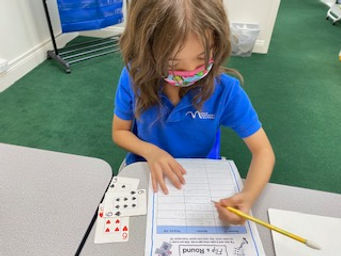## Ms. Dawn

### Target 1​

###### Lesson Type:

Continuation

Number Operation

:

Integer Composition

Use place-value understandings to round whole numbers to the nearest ten or 100.

###### 1:

Use understanding of how to round two-digit numbers and expand the strategy to three-digit numbers.

###### 2:

Understand that the previous place (one place smaller) is the key digit to evaluate to determine whether a number is rounded up or down.

3rd

###### Vocabulary:

Round, Ones, Tens, Hundreds, Nearest

Activities:

1. Students played a game called "Flip and Round." They flipped three cards to create a three-digit number. Then, they rounded their flipped number to the nearest tens and then to the nearest hundreds.

2. Students rounded to the nearest tens, hundreds, and even thousands in a maze.The target was to work their way from the "Start" box to the "Finish" box.### Home Exploration

###### Challenge Problem:

Get a deck of cards and flip over 4 or more cards. Have your child create a number using the playing cards and then round to the nearest tens and hundreds.

###### Guiding Questions:

What is the rule when rounding?## Absent Students:

### Target 2

:

###### 1:

Understand equivalent measurements.

3rd

###### Vocabulary:

Equivalent, Inches, Feet, Yards, Miles, Millimeter, Centimeter, Meter, Kilometer, Quarts, Pints, Cups, Pounds, Ounces,

Activities:

1. Students played a game that dealt with matching the equivalent measurements. There were paper cards with measurements on it faced down. When it was their turn, the student flipped over two cards to try and find a match. Once they found an equivalent measurement match, they kept their pair.

2. Students were given a variety of measurement cards and needed to find what is equivalent (ex. 8ft. = ______ in.) Students took turns until all the cards were completed.

3. Students used a measurement chart to help them complete a unit conversion maze.### Home Exploration

Have students convert measurements in the kitchen or when you measure or build something. Ask how many quarts are in the gallon of milk that's in the refrigerator.

How many cups are in a pint? How many quarts make a gallon? How many centimeters in a meter?

###### Guiding Questions:### Target 3

:

###### Vocabulary:

Activities:### Home Exploration